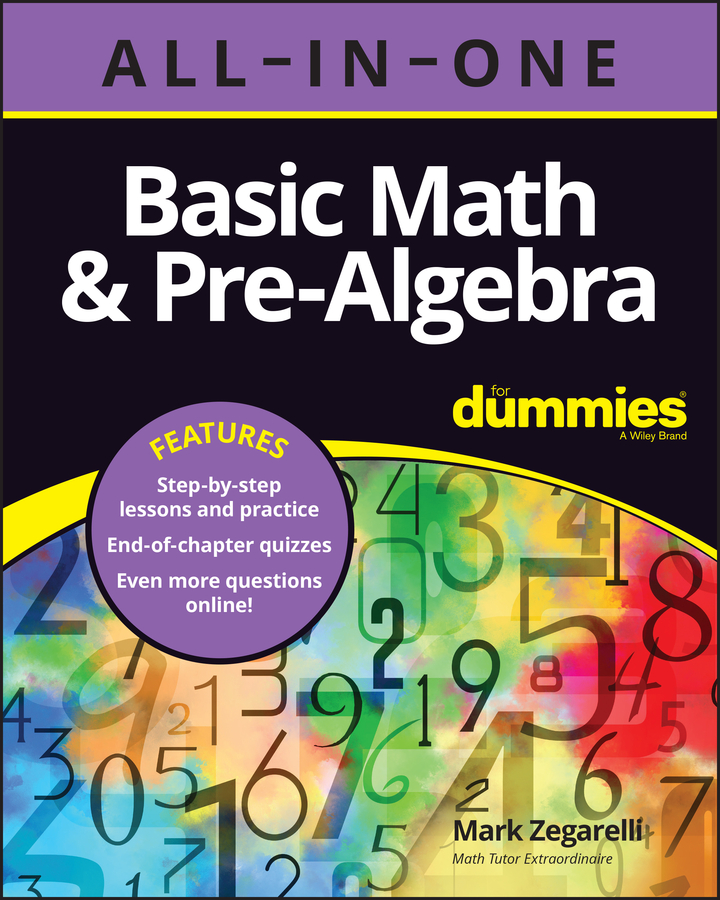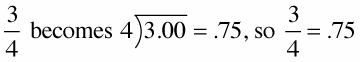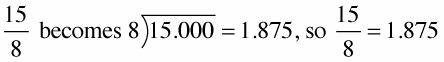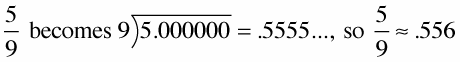##### Basic Math & Pre-Algebra All-in-One For Dummies (+ Chapter Quizzes Online)You can convert all fractions to decimals. The decimal forms of rational numbers either end or repeat a pattern. To convert fractions to decimals you just divide the top by the bottom — divide the numerator by the denominator — and if the division doesn't come out evenly, you can stop after a certain number of decimal places and round off.

To convert fractions to decimals:

1. If the fraction is a mixed number, change it to an improper fraction.

2. Divide the numerator by the denominator.

3. If the division doesn't come out evenly, round the decimal off.

Here are some examples:

The fraction 3/4: Divide 3 by 4, and you end up with the decimal .75.The fraction 15/8: Divide 15 by 8, and you end up with the decimal 1.875.Of course, these examples have divided evenly so far, but if the division doesn’t come out evenly, you can stop after a certain number of decimal places and round off.

The fraction 5/9: Divide 5/9 to get the decimal .555. If you choose to round to three decimal places, as in this example, you get an approximate answer, .556.